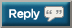# Thread: Let's OHP 2,020,000 lbs in 2020

1. +1455 yesterday as some assistance = 3911882. +1455 yesterday as some assistance = 391188
391,188

OHP

135 x 5
135 x 5
135 x 5

2,025

391,188 + 2,025

393,2133. 391,188

OHP

135 x 5
135 x 5
135 x 5

2,025

391,188 + 2,025

393,213
+ 2,170 = 395,3834. Terrible workout today, not as bad as my 1 rep workout last year, but close second.
During first warm up for squat, the bar was a huge 30Kg, I got a stinging pain in my left leg below the knee, a few more reps didn't fix it, so abandoned squat, DL, hypers and all the stuff I'd planned. But I did manage to do a mini OHP session before hitting the showers

+3,016 =398,339

The next contributor should get to bust the 400,000 mark.5. The next contributor should get to bust the 400,000 mark.
Wooop I get the honour

398339 + 1901 = 4002406. Arnold press:

400,240 + 3200 = 403,4407. Arnold press:

400,240 + 3200 = 403,440
Included my warm up sets in the total this time.

403,400 + 8730 = 412,1308. Arnold press:

400,240 + 3200 = 403,440
4488 + 403440 = 4079289. Correcting total:

403,440 + 8730 + 4488 = 416,65810. +1980 = 41863811.12. DB & APress 4 x 19 x (30 x 2) = 4560

4560 + 419808 = 42436813. Time to come clean - all my lifts here have been 'cheaty OHP' as recommended by Greg Nuckols. Hence by PB for strict OHP is still 5 x 40kg, because I've been using leg drive for remaining reps as soon as I feel 1-2 reps shy of failure.

7 x 40kg
7 x 40kg
9 x 40kg (AMRAP)

920kg (2028lb)

2028 + 419808 = 42183614. Barbell strict OHP, was supposed to have been a bigger "A" one, I was just too tired, so did a small "B" one instead
20*10, 30*10, 40*10, 50*7, 62.5*1, 61*1 (I'd carried some fractional plates so may as well use them for a rep)

+3,022 =424,85815. Correct number as one was missed above (see post 132 - 133):-

42941816. Correct number as one was missed above (see post 132 - 133):-

42941817. .. I've been using leg drive for remaining reps as soon as I feel 1-2 reps shy of failure...
Perfectly acceptable within this thread's rules
All freeweight overhead presses count: standing or seated, barbell or dumbbell, strict press or push press...18. ... I've been using leg drive for remaining reps as soon as I feel 1-2 reps shy of failure....
I end all my OHP workouts with push presses, but not until I form fail with the strict ones. I highly recommend doing it that way.19. DB & APress 4 x 15 x (35 x 2) = 4200
DB press 1 x 8 x (2x2) = 32

429418 + 4200 + 32 = 43365020. DB & APress 4 x 15 x (35 x 2) = 4200
DB press 1 x 8 x (2x2) = 32

429418 + 4200 + 32 = 433650
5489 + 433650 = 43941921. + 7765 = 447,18422. Behind a bit so need to catch up...

+ 17,804 = 464,98823. Behind a bit so need to catch up...

+ 17,804 = 464,988
+ 2,260 = 467,24824. Barbell strict OHP
+8,015 =475,26325. + 2,420 = 477,68326. DB & APress 4 x 20 x (30 x 2) = 4800; 1 x 17 x (.5 x 2) = 17

4817 + 477,683 = 482,50027. DB & APress 4 x 20 x (30 x 2) = 4800; 1 x 17 x (.5 x 2) = 17

4817 + 477,683 = 482,500
+ 3080 = 48558028. 3740 + 485580 = 48932029. Top set of 5 @ 160
Back down work 120 - 5x10

489,320 + 9870 = 499,190

Next poster breaks 500k!30. 4 sets of 7 (final set was AMRAP but still got 7) @ 42.5kg = 1190kg (2623lb)

2623 + 499,190 = 501,813#### Posting Permissions

• You may not post new threads
• You may not post replies
• You may not post attachments
• You may not edit your posts